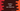# How to read a user input list in python## Introduction:

Sometimes we need to read a list of inputs from the user. For example, if our program needs a list of strings as an input from the user, we will have to ask the user to enter these values. We can get them one by one or all in one go. In this post, I will show you how to handle this situation in Python.

## Reading the inputs one by one:

Let’s read the inputs one by one. Our application will ask the user to enter the list, the user will enter each input one by one and finally, our application will print out the list entered by the user.

``````num_list = []

count = int(input("Enter the total count of elements : "))ff

for i in range(0,count):
num_list.append(int(input()))

print(num_list)``````

In this example,

• numlist_ is an empty list. We will add all the elements to this list.
• count is for storing the total count of elements. The second line is asking the user to enter the total number of elements and it stores this value in the count variable.
• Using one for loop, we are reading the elements one by one from the user. These elements are appended to the empty list numlist_.
• Finally, we are printing out the list numlist_.

If you run this program, it will look like as below:

This method will work just fine but the main problem is that the user will have to enter each number one by one, which may not be suitable for your project.

## Reading the inputs from a single line:

Let’s try to read the inputs in one go. The user will enter the elements separated by a space and our program will read them and put them in a list.

``````elements = input("Enter all elements separated by space : ")

num_list = list(map(int,elements.strip().split()))

print(num_list)``````

Here, we are not asking the user for the total counts. The user can enter as many elements as he wants. The program will read all entered numbers as a string and put it in the elements variable. We are creating one map by splitting all elements of the input string and we are converting this map to a list. The final list is stored in the numlist_ variable.